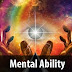## Recent Posts

1. What is the angle of rotation of the minute hand of a clock when the time is changed from 12'O'clock to 5:10?
[a] 148
[b] 160
[c] 150
[d] 155

2. If rectangle is called circle, circle is called point and point is called triangle, what is the shape of wheel?
[a] Circle
[b] Point
[c] Rectangle
[d] Triangle

3. A is the parent of C and D. F is the only daughter of K whose husband is A. D,C and F are siblings. How is D related to K?
[a] Mother
[b] Son
[c] Daughter
[d] Father

4. Which is the wrong number in the series:
11, 2, 21, 3, 32, 4, 41, 5, 51, 6

[a] 4
[b] 41
[c] 32
[d] 21

5. DXP, GWN, JVL, MUJ, ?
[a] PTG
[b] PTH
[c] OTH
[d] OTG

6. Which is the one that does not belong to the group?
[a] Knowledge
[b] Learn
[c] Study

7. Find out the missing number:
60, 64, 32, 36, 18, 22, ___
[a] 20
[b] 12
[c] 11
[d] 24

8. 'B' is A's sister, 'C' is B's mother. 'D' is C's father. 'E' is D's mother. How is 'B' related to 'E'?
[a] Great grand-daughter
[b] Grand son
[c] Great grandson
[d] Grand daughter

9. Five students are sitting in a row. 'T' is in the north of 'Z'. 'M' is on the left of 'Z' but is on the right of 'L'. 'T' is on the left of 'Q'. Who is sitting first from the left?
[a] Z
[b] Q
[c] T
[d] L

10.  Which is the one that does not belong to the group?
Tea, Rubber, Cardomom, Chalk, Cinchona
[a] Tea
[b] Rubber
[c] Cardomom
[d] Chalk

Kerala PSC - Mental Ability 06 (Reasoning)Reviewed by Santhosh Nair on 8:44 PM Rating: 5